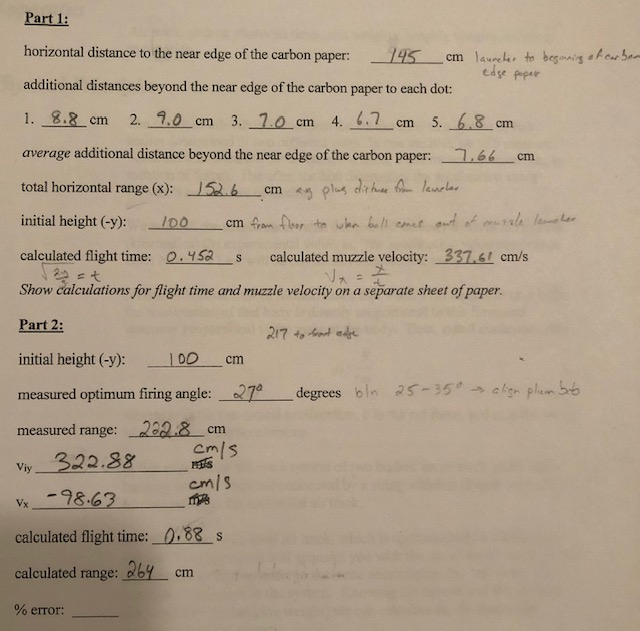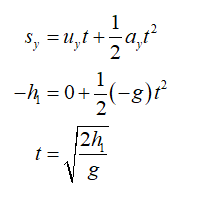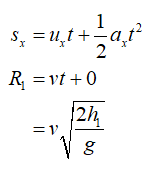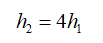# Part 1:horizontal distance to the near edge of the carbon paper:145 cm launeher to begmaing et cr bemedse peperadditional distances beyond the near edge of the carbon paper to each dot:1. 8.8 cm2. 9.0 cm 3. 1.0 cm 4. 6.75. 6.8 cmстстaverage additional distance beyond the near edge of the carbon paper:.66cmtotal horizontal range (x):leurler52.6cm s plus dr huinitial height (-y):cm fram floor to ukn bull cmer ut of murale lamme hercalculated flight time:0.452calculated muzzle velocity: 337.61 cm/sShow calculations for flight time and muzzle velocity on a separate sheet of paper.Part 2:217 to front edgeinitial height (-y):100cт> algn plum bb27°degrees bln 25-350-measured optimum firing angle:measured range:222.8 cmCm/s322.88Viycm/s-98.63Vx0.88 scalculated flight time:calculated range: 264 cm% error:

Question
7 views

By what numerical factor will the range increase if you launch the ball horizontally from a height which is four times greater than in the lab? Explain your answer.help_outlineImage TranscriptionclosePart 1: horizontal distance to the near edge of the carbon paper: 145 cm launeher to begmaing et cr bem edse peper additional distances beyond the near edge of the carbon paper to each dot: 1. 8.8 cm 2. 9.0 cm 3. 1.0 cm 4. 6.7 5. 6.8 cm ст ст average additional distance beyond the near edge of the carbon paper: .66 cm total horizontal range (x): leurler 52.6 cm s plus dr hu initial height (-y): cm fram floor to ukn bull cmer ut of murale lamme her calculated flight time: 0.452 calculated muzzle velocity: 337.61 cm/s Show calculations for flight time and muzzle velocity on a separate sheet of paper. Part 2: 217 to front edge initial height (-y): 100 cт > algn plum bb 27° degrees bln 25-350- measured optimum firing angle: measured range:222.8 cm Cm/s 322.88 Viy cm/s -98.63 Vx 0.88 s calculated flight time: calculated range: 264 cm % error: fullscreen
check_circle

Step 1

Let v be the horizontal velocity with which the ball is launched, 1 be the height in the lab, h2 be the height in actual, R1 be the range of the ball when it is launched in the lab, R2 be the range when it is fired in actual.

Write the second kinematic equation of motion for the ball in the vertical direction.Step 2

Write the second kinematic equation of motion for the ball in the horizontal direction and substitute the known values.Step 3

Write the relation between both the heights....

### Want to see the full answer?

See Solution

#### Want to see this answer and more?

Solutions are written by subject experts who are available 24/7. Questions are typically answered within 1 hour.*

See Solution
*Response times may vary by subject and question.
Tagged in

### Physics